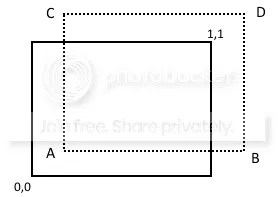Noise functions can be used for all sorts of things in graphic games, like backgrounds, and 3D terrain height maps.

In this post, I’m going to look at one specific use – animated textures, such as flowing water – and how difficult this is to achieve.

It involves seamlessly tiling images, though, which is really really cool (and useful).

It also requires shaders, so it’s not for beginners. (Sorry, I don’t like to exclude anybody).

I know I’ve talked about noise before, but I’ll explain it again anyway.

Perlin noise (named after the guy who created it) is a formula that calculates a number between -0.5 and +0.5 based on the parameter(s) you give it.

So noise(0.1) = 0.09 and noise(0.2) = 0.14

It sounds like random numbers, with two differences. First, noise(0.1) will always give you 0.09 (wait for it, don’t ask what’s the point, just yet!).

Second, and most important, the numbers are correlated (ie depend on) the numbers around them. So noise(0.10001) will give an answer very close to 0.09, because 0.1 is very close to 0.10001. However, noise(0.8) could be very different, because 0.8 is a long way from 0.1.

Let me use an example to explain.

Imagine a landscape with rolling hills and valleys. Suppose you can measure the height of the landscape at any place in the landscape. If you measure the height at a series of points one centimetre apart, you will get a very smooth result. But if you measure the height at points one kilometer apart, you will get much more variation in the heights from one point to the next.

Perlin noise gives you a landscape of heights, all between -0.5 and +0.5. If you choose a series of points close together, you will get results that vary smoothly, but if you make them further apart, the results will be bumpier.

So here is one example of using noise to create rolling hills. Code is here.### Animating a noisy pattern

Suppose I want to create the illusion of water flowing. I can take code like that in the Codea demo noise app, overlay a transparent blue image, and it looks pretty good.

Well, maybe.

First, it looks blocky, because the program divides the screen into little squares and gives all the pixels in each square the same value. The reason is clear, from the second problem, speed. Even if you only calculate noise for blocks of pixels, and not for individual pixels, the program only runs at about 30fps on my iPad 3.

30 fps with nothing else on the screen. Hmmmm. That’s no good. Doesn’t leave room to add any kind of a game.

### Finding faster noise

So I went looking for faster noise, and discovered that Perlin had developed a faster algorithm later, called simplex noise. I also found shader code for it (you are not seriously going to try running these formulae in Lua!).

The good news is that simplex noise is much faster.

The bad news is that if you then model noise for individual pixels, rather than blocks, you are back to 30fps again. So we’ve only solved half of the problem.

So I decided to try something different.

Suppose you start by drawing a big image, bigger than the screen (or whatever area you are animating). You fill this image with noise, and scroll it across the screen, and when you get to the end, you start again at the beginning. The result should be way faster than rendering noise in real time, and if the image is large enough, the viewer won’t notice it repeating.

Except for one thing.

The image is not seamless – in other words, if you lay two copies next to each other, you can clearly see the join, because the patterns don’t match at the edges. So when you scroll, you will see the join every time the image restarts.

Actually, there’s another question. How do you scroll an image endlessly, to start with?

### Scrolling an image endlessly

By adapting this technique. That was easy.

At this stage, I tested the speed if I created an image (say) 50% larger than the screen, and scrolled it endlessly.

FPS =60. Speed problem solved.

Now what about that nasty join every time the image wraps around to the start again?

### Making an image seamless

The answer to this can be quite simple.

Suppose the tiled image is the solid rectangle below, and our shader is drawing at the point A shown.Then we interpolate between the noise value at A and the points B, C and D shown above. This means that as you approach an edge, it gets closer to the value at the opposite edge, creating a seamless fit.

```    //create scaled copy of texture position
//noise is taken from the position (x,y) * scale + offset
highp vec2 p = offset+scale*vec2(vTexCoord.x,vTexCoord.y);
//interpolate
float A = simplexNoise2(p);
float B = simplexNoise2(vec2(p.x+scale,p.y));
float C = simplexNoise2(vec2(p.x,p.y+scale));
float D = simplexNoise2(p+scale);
float xx = 1.0 - vTexCoord.x;
float yy = 1.0 - vTexCoord.y;
float AB = mix(A,B,xx); //interpolate A,B on x axis
float CD = mix(C,D,xx); //interpolate C,D on x axis
float u = mix(AB,CD,yy); //interpolate results on y axis
//because noise goes from -1 to +1, and we want it 0-1
u=(u+1.0)/2.0;
gl_FragColor = vec4(u,u,u,1.0);
```

My final code is here.